Advanced Multimedia Processing Lab -- Projects -- Hand-Drawn Sketch Retrieval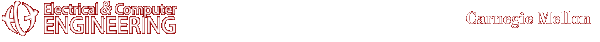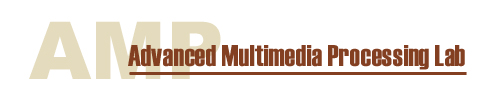#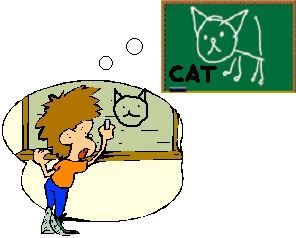Howard Wing Ho Leung wingho@andrew.cmu.edu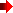Top of this page

 Motivation and Goal

Consider the scenario in the classroom: the lecture notes written by the teacher on the whiteboard can be captured electronically by a pen-based device such as "mimio" and later students can retrieve relevant lecture materials from the hand-drawn sketch database by sketching a query drawing. There is no need for text recognition for the hand-written text therefore OCR errors are avoided. The sketches can be free-form or abstract drawings since it is not necessary to understand the context of the sketches.Top of this page

System Overview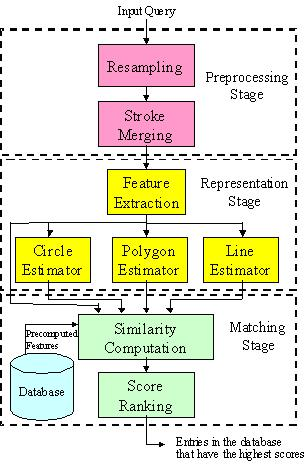Figure 1. System overview of the sketch retrieval system

Resampling

The input query, a single stroke or multiple strokes, is resampled to 256 points according to the arc-length. When the input query consists of multiple strokes, the number of resample points are distributed according to the proportion of the arc-length of each stroke. This resampling process reduces inconsistencies due to different writing speed.

Stroke Merging

In order to account for different styles of drawing strokes, two strokes are merged into one stroke if the distance between a start/end point of a stroke and a start/end point of the other stroke is small, as shown in Figure 2. However, if the start and end points of the same stroke are already very close, i.e., a closed loop, then this stroke should not be merged with other strokes because the location of the start and end points of a closed-loop stroke can be arbitrary, as shown in Figure 3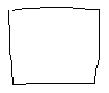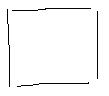(a) (b)

Figure 2. A sketch formed by different combination of strokes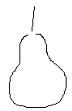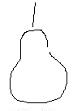(a) (b)

Figure 3. A sketch containing a closed-loop stroke formed by different location of start and end points

Feature Extraction

Some basic features are extracted from each stroke such as the center of the stroke, the perimeter, the area, the convex hull, etc. Other features are computed by combining the basic features. For example:

 1) Perimeter efficiency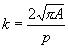, where A is the area and p is the perimeter. 2) The ratio between the area formed by the original stroke and the area of its convex hull. 3) The ratio between the number of points of the convex hull and the number of point of the original stroke samples.

These features are used for the shape estimators to determine the likelihood that each stroke falls in each basic shape type: line, circle and polygon. A likelihood measure that takes the value between 0 and 1 is assigned for each stroke with respect to each shape type.  For example: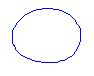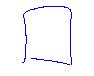(a) 0.8536 (b) 0.6806 (c) 0.273

Figure 4. Some sketches and their likelihood values from the circle estimator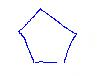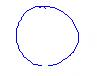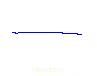(a) 0.9772 (b) 0.5390 (c) 0

Figure 5. Some sketches and their likelihood values from the polygon estimator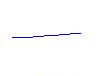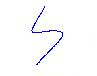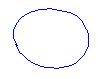(a) 0.9953 (b) 0.4958 (c) 0

Figure 6. Some sketches and their likelihood values from the line estimator

Matching Score between two strokes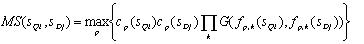where sQi is the i-th stroke of the query, sDj is the j-th stroke of the sketch from the database, p is the shape type which can be line, circle, polygon or non-basic type, cp(s) is the likelihood of the estimator of the shape type p for the stroke s; fp,k(s) is the k-th feature from the estimator of the shape type p about stroke s; G(f1,f2) is the similarity measure between features f1 and f2. The non-basic type is one of the shape types and its confidence value is derived from the confidence values of the other basic shape types. The confidence value of the non-basic type is high when the confidence values of all the basic shape types are low. The features used for the similarity measure for the non-basic type are taken from each of the estimators of the basic shape type.

Matching Score between two sketches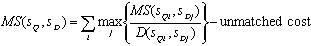where sQ is the query sketch, sD is the sketch from the database, is a distance measure between the i-th stroke of the query, and the j-th stroke of the sketch from the database.

Experiments and Results

We perform an experiment to analyze the retrieval performance based on our approach. Our database consists of 35 categories of free-form sketches. Initially, for each sketch we have 20 repetitions made by 4 different people to account for the different drawing styles. Eight months later, the same people are asked to redraw the sketches 5 more times per person. Figure 7 shows a few examples of these sketches in the database. Figure 8 shows example sketches drawn by the same person at two different time instants (8 months apart). There is significant variation in the drawings even if they are drawn by the same user. We then compute the matching scores between a query and the sketches from the database. Based on the rank of those sketches that fall in the same category, we plot the precision and recall graph in order to analyze the retrieval performance. The result is shown by the two curves in Figure 9. The top curve corresponds to the retrieval performance when each of the sketches from the initial collection is used as the query. The bottom curve corresponds to the retrieval performance when each of the sketches drawn 8 months later is used as the query, matching with the initial collection of the sketch database. At the recall rate of 0.5, the precision rate is higher than 0.8 for both cases. This means that as we keep retrieving sketches from the database, when 50% of the sketches from the same category as the query (relevant sketches) are retrieved, 80% of the total retrieved sketches are relevant sketches. It can also be seen from Figure 9 that the two sets of query yields similar retrieval performance, showing that our system is robust to variation over time.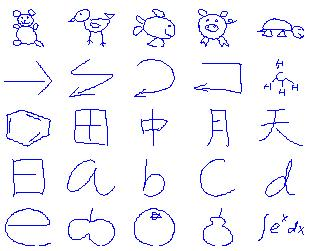Figure 7. Some examples of the sketches in the database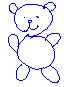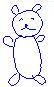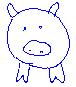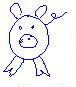(a) initial collection (b) 8 months later (c) initial collection (d) 8 months later

Figure 8. Example sketches drawn by the same user at two different time instants (8 months apart)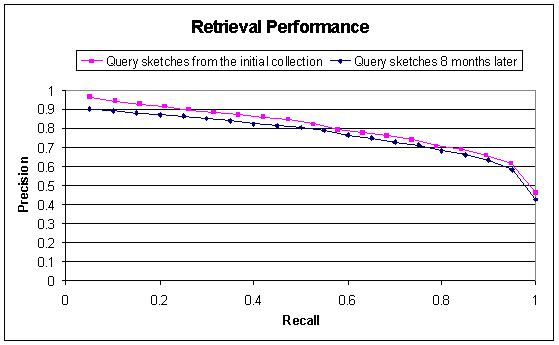Figure 9. Retrieval performanceTop of this pageTop of this page

Any suggestions or comments are welcome. Please send them to Howard LeungTop of this page